Related Articles
Batch statement in Cassandra
• Last Updated : 09 Dec, 2019

In this article, we will discuss the BATCH statement, good and misuse of BATCH statement and how we can use the BATCH statement in single and multiple partitions in Cassandra which is supported by Cassandra Query Language (CQL). let’s discuss one by one.

We can use the BATCH statement in single and multiple partitions which ensure atomicity for both the operations.

So, let’s take an example.
First, we create a table namely as bank_emp_data which has Id, Name, emp_start_date, emp_end_date fields. Let’s have a look.

```CREATE TABLE bank_emp_data(
E_id int,
Name text,
emp_start_date date,
emp_end_date date,
PRIMARY KEY (E_id, Name)
); ```

Now, here we are going to write data into bank_emp_data table without using BATCH statement. Let’s have a look.

```INSERT INTO bank_emp_data(E_id, Name, emp_start_date, emp_end_date)
VALUES (1005, 'Ashish', '2019-12-05', '2025-12-05');

INSERT INTO bank_emp_data(E_id, Name, emp_start_date)
VALUES (1006, 'Rana', '2019-12-05');

INSERT INTO bank_emp_data(E_id, Name, emp_end_date)
VALUES (1007, 'shiv', '2020-12-05'); ```

To see the output used the following CQL query.

```Select *
From bank_emp_data; ```

Output: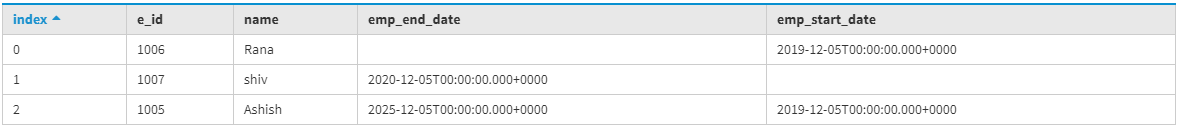Now, to update an existing row in bank_emp_data table used the following CQL query given below.

```Update bank_emp_data set emp_start_date = '2019-12-05'
where E_id = 1007 and Name= 'shiv';  ```

To see the updated row used the following CQL query.

```Select *
from bank_emp_data; ```

Output: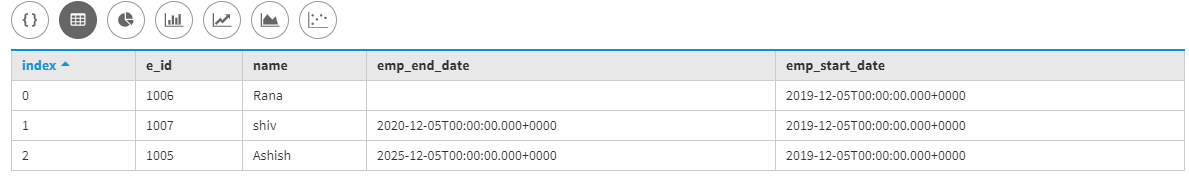Now, here we are using BATCH statement and it also shows how we can good use of BATCH statement. Let’s have a look.

```BEGIN BATCH
INSERT INTO bank_emp_data(E_id, Name, emp_start_date, emp_end_date)
VALUES (1005, 'Ashish', '2019-12-05', '2025-12-05');

INSERT INTO bank_emp_data(E_id, Name, emp_start_date)
VALUES (1006, 'Rana', '2019-12-05');

INSERT INTO bank_emp_data(E_id, Name, emp_end_date)
VALUES (1007, 'shiv', '2020-12-05');

Update bank_emp_data set emp_start_date = '2019-12-05'
where E_id = 1007 and Name= 'shiv';
APPLY BATCH; ```

Now, let’s see the output of bank_emp_data using BATCH statement.

```Select *
from bank_emp_data; ```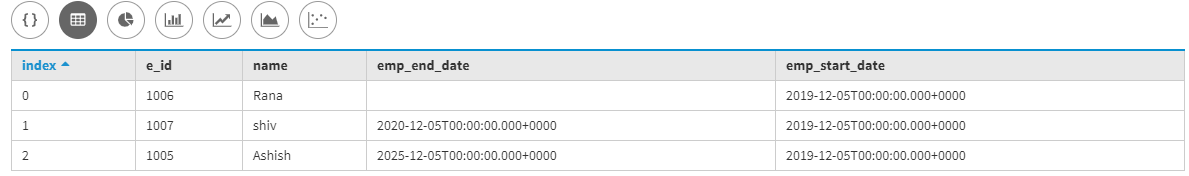Multiple partition batch:
We can write data into different tables by using the BATCH statement.

Let’s understand with an example.

Table: Emp_data

```create table Emp_data(
E_id int primary key,
Name text,
city text
); ```

Table: Emp_data_copy

```create table Emp_data_copy(
Name text primary key,
E_id int
); ```

Now, we are using BATCH to insert data into different tables.

```BEGIN BATCH
INSERT INTO Emp_data (E_id, Name, city)
VALUES (101, 'Ashish', 'california');

INSERT INTO Emp_data_copy (Name, E_id)
VALUES ('Ashish', 101);
APPLY BATCH; ```

Now, let’s see the output of Emp_data table.

```SELECT *
FROM Emp_data;```

Output: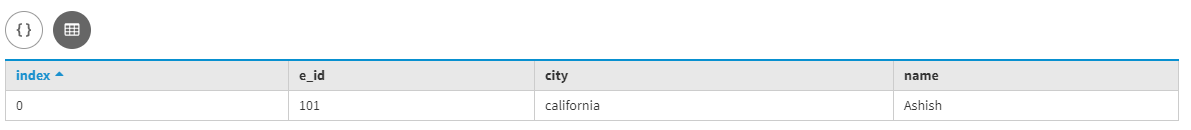Now, let’s see the output of the Emp_data_copy table.

```SELECT *
FROM Emp_data_copy;```

Output: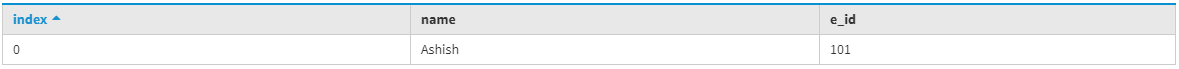Attention reader! Don’t stop learning now. Get hold of all the important CS Theory concepts for SDE interviews with the CS Theory Course at a student-friendly price and become industry ready.

My Personal Notes arrow_drop_up